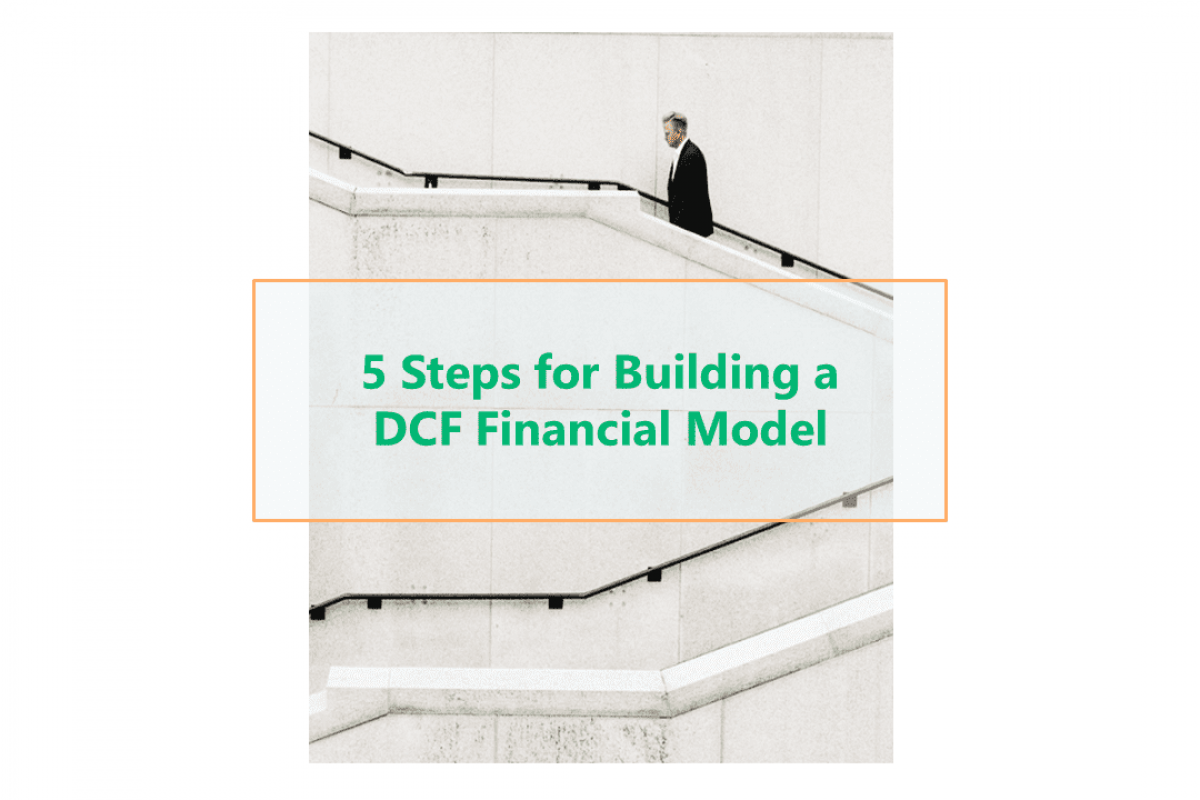# 5 Steps for Building a DCF Financial ModelKeyskillset
16
January
2020### What is DCF Financial Model?

A discounted cash flow model (“DCF model”) is a specific type of financial model used to value a business. It values a company by forecasting its’ cash flows. Then, it discounts the cash flows to arrive at the current, present value.

Valuing companies using the DCF is a core skill for investment bankers, private equity, equity research analysts and investors.

Let’s see how to build a DCF financial model step by step.

#### Step 1. Derive historical information from financial statements.

There are three main financial statements every company has: Income Statement, Balance Sheet and Cash Flow Statement. You can learn more about it in our article “Using Financial Statements in Financial Modeling”.

The first step in any financial model is to derive necessary information from financial statements. You will create historical data in the spreadsheet with the model. Then you will need to calculate the necessary totals.

In our example, we need to calculate Unlevered Free Cash Flow (UFCF). So first, we derive all the necessary information for that from financial statements. Then, we calculate UFCF.

#### What is Unlevered Free Cash Flow?

Unlevered Free Cash Flow is the gross free cash flow generated by a company. Leverage is another name for debt, and if cash flows are levered, that means they are net of interest payments. The formula for UFCF is:

Unlevered FCF = EBITDA – CapEx – Working Capital – Tax Expense
Or
Unlevered FCF = Net Income + D&A – Capex – Working Capital.

We created our sample model to calculate UFCF by using the Excel shortcut Auto-sum Ctrl + Shift + ‘T‘.

#### Step 2. Calculate historical value drivers

Next, we need to create a list of historical value drivers to use in our model.

You can find the list of value drivers for our sample model in the screenshots below.

#### Step 3. Make assumptions for the projected value drivers

For our sample model, we assumed that the Revenue Growth (%) in 2019 is the same as in 2018. Then, it goes down by 0.5% each following Projected Year, so we will use the step here.

For the other Value Drivers in 2019, we will take the average of the previous three years. For the following years, we assume they will stay the same, so we’ll use a flatline here.

To learn better how to build a flatline or steps in assumptions for financial models, read our article “Top 5 rules for Formatting Financial Models with Excel tricks”.

#### Step 4. Calculate projected line items for the model.

The next step is to calculate the projected line items. They are based on historical data and projected value drivers we would calculate in the previous step.

To remember the first fours steps of DCF financial modeling better, think of the four quadrants. You would fill them one after another (see the picture below).

#### Step 5. Calculate Equity Value per share

The final step and final goal of DCF modeling are to find equity value per share. You can see the big picture of the necessary calculations on the sample model below. More descriptive calculation you can find in our article “Equity Value per Share calculation in DCF models”.

### What to remember about DCF?

Here are some of the points that you need to remember:

1. Discounted cash flow (DCF) helps determine the value of an investment based on its future cash flows.
2. The present value of expected future cash flows uses a discount rate to calculate the discounted cash flow (DCF).
3. There are limitations in terms of using DCF. Primarily that it relies on estimations on future cash flows. That could prove to be inaccurate.
4. To remember the logic of building DCF or any other financial model think of four quadrants. You need to build them one after another. Use historical data from the financial statement. Then, go to historical value drivers followed by projected value drivers. Choose projected line items. Finally, build the necessary calculation on top of that.
5. Play KeySkillset educational game Financial Modeling and follow the logic. Learn how to build a financial model hands-on step by step.